you are here:/ News & Insights / Engineering Advantage Blog / Random Data Analysis Part 8: Auto & Cross Correlation

# Random Data Analysis Part 8: Auto & Cross CorrelationMay 4, 2016 By: Jonathan Dudley

In Part 7 of this series, the use of Short-Time Fourier to obtain amplitude, time, and frequency content was discussed. This post will introduce to the concept of Cross-Correlation and will discuss some benefits of performing this type of analysis.

Cross-correlation analysis can be used to help determine how strongly two signals in the time-domain are related. If the signals are identical the correlation coefficient is unity and if they are unrelated the correlation coefficient is zero. If the signals are identical except that the phase is shifted by exactly 180 degrees, then the correlation coefficient is -1. When two independent signals are compared in the time domain, the procedure is known as cross-correlation. Autocorrelation is a special case of cross-correlation where the same signal is compared to itself. The auto correlation coefficient of a signal x(t) is given as: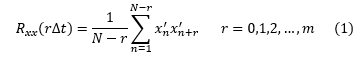The time lag, or separation between points, is given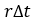. The maximum number of lags is given by r. The cross-correlation of two signals x(t) is y(t) and is then given by Equation 2: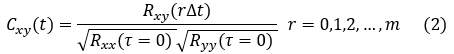The cross-correlation performed in the time domain is a computationally inefficient approach which may be computed faster taking advantage of the FFT. The enhanced correlation is written by convolving the first function, g(t),with the time-reverse of the second function given by h(t):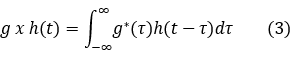where the * is the complex conjugate and the x indicates the cross-correlation. This may be implemented with the following routine using Python:

def xcorrNorm( y, y2, fs, maxlags):

import numpy as np

####### Cross Correlation via FFT ###############

fs=float(fs)

y = y - np.mean(y) # sig 1

y2 = y2 - np.mean(y2) # sig 2

N = len(y)

sp = np.fft.rfft(y) # compute FFT transform of 1st signal

sp2 = np.fft.rfft(y2) # compute FFT of 2nd sig

sp = np.conj(sp) # complex conjugate of 2nd sig

rho = np.fft.irfft( sp*sp2 ) # inverse transform of the convolution

rho = np.fft.fftshift(rho) # shift fft so 0 is centered

rho = rho / (np.std(y) * np.std(y2)) / N  # normalize

tlag = np.int32(np.linspace(-len(rho)/2,len(rho)/2,len(rho))) / fs # Get lags

rho = rho[(N/2) - maxlags : (N/2) + maxlags] # extract maxlag data

tlag = tlag[(N/2) - maxlags : (N/2) + maxlags]  # extract maxlag data

return tlag, rho

Figure 1: Auto & Cross Correlation Coefficients for the Time Series, presented in previous posts

## rda8_1.pngFigure 1 represents the cross and auto correlation of given pressure signals discussed in the previous posts. Referring to Figure 1, we know the offset from the leading edge to x/L=0.25, we may estimate the upstream speed of the propagating wave by looking at the offset of the corresponding peaks of these curves. In this example we obtain: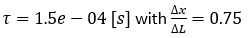(L=0.0762 [m]) and a velocity of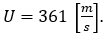Also, analogous to comparing peaks for the cross-coherence in Part 7 of this series, we see here that the cross-correlations exhibit well-correlated peaks indicating what is sensed at the aft wall is also strongly correlated with the pressure signal upstream. As expected, the black line exhibits a peak of unity at zero lag since this is the auto-correlation. In my next and final post in this series, I will discuss the use of two point statistics using turbulent velocity and fluctuating pressures. If you have any insights on this approach, I'd enjoy hearing them in the comments!